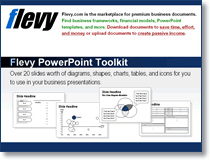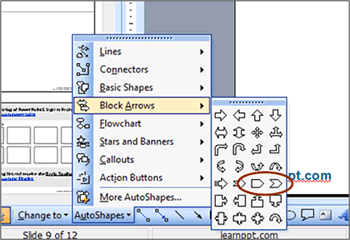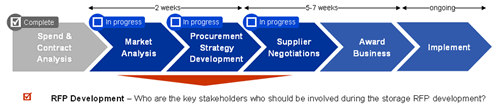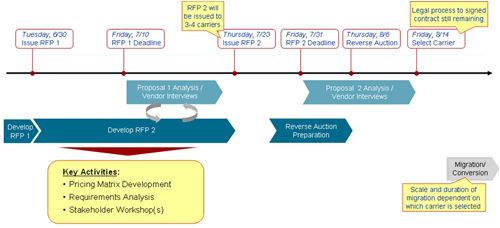×

# Receive our FREE PowerPoint ToolkitThe Flevy PowerPoint Toolkit contains over 50+ slides worth of diagrams, shapes, charts, tables, and icons for you to use in your business presentations.

The following is an except from my eBook, Become a PowerPoint Guru.  This is the introduction to Chapter 8, Approach.

——

You will find most executive presentations have an Approach slide somewhere in the beginning.  The Approach shows a simple, intuitive diagram of how a certain initiative is laid out.  An example would be phases of a project (e.g. 1. Define the Problem, 2. Generate Hypotheses, 3. Conduct Research, etc.).  This chapter will cover how to quickly create an Approach Diagram and leverage it throughout your presentation for consistency.

First off, an Approach is nothing more than a sequence of steps.  A very basic example is a series of phases.  See below for an illustrative example:

Almost every presentation produced by a consulting firm has a diagram like the one above.  PowerPoint also makes it very easy to draw a diagram like that, as it is comprised of 2 PowerPoint AutoShapes, the Pentagon (used to start the Approach) and the Chevron (used for all other subsequent steps).  You can find both these AutoShapes under ‘Block Arrows.’  Because these two shapes are so widely used, you may have noticed I have added them as shortcuts in my PowerPoint Set-up (Chapter 2).  See the screenshot belowWhen you construct this diagram, always use a pentagon to start the Approach.  The flat edge on the right delineates that this is the start of the Approach.  When creating the diagram initially, don’t worry about sizing things correctly.  Once you create the full, X-Step Approach diagram, select all the shapes and group them (i.e. Ctrl+G).  This will allow you to easily resize the diagram as a cohesive unit.

Building on the Approach Diagram

There are many small things you can add on to this simple diagram to add additional insights and depth.  For instance, adding numbered circles furthers emphasizes the sequence of steps.  You can highlight a specific chevron to indicate ‘current phase/step.’  Furthermore, you can add an element of timing using a parallel arrow—or, by resizing the lengths of the chevrons.  The examples below illustrate Approach diagrams with added embellishments.Example 1Example 2 (Approach-Gantt hybrid)

Beyond the Basic

So, a series of steps not cutting it for your approach?  There are various deviations from the basic Approach Diagram outlined above.  Here are the most common:

• Steps in parallel instead of series
• Steps that are cyclical – i.e. the last step returns to step 1
• Steps that converge

—–

I’ll post the rest of this chapter in a future blog post.

Tagged with: